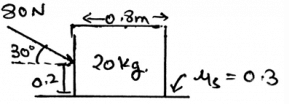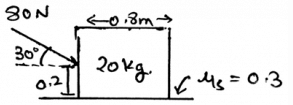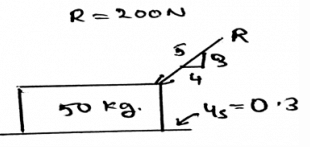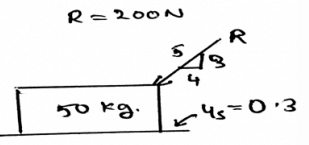Test: Work

# Test: Work

Test Description

## 30 Questions MCQ Test Engineering Mechanics | Test: Work

Test: Work for Mechanical Engineering 2022 is part of Engineering Mechanics preparation. The Test: Work questions and answers have been prepared according to the Mechanical Engineering exam syllabus.The Test: Work MCQs are made for Mechanical Engineering 2022 Exam. Find important definitions, questions, notes, meanings, examples, exercises, MCQs and online tests for Test: Work below.
Solutions of Test: Work questions in English are available as part of our Engineering Mechanics for Mechanical Engineering & Test: Work solutions in Hindi for Engineering Mechanics course. Download more important topics, notes, lectures and mock test series for Mechanical Engineering Exam by signing up for free. Attempt Test: Work | 30 questions in 60 minutes | Mock test for Mechanical Engineering preparation | Free important questions MCQ to study Engineering Mechanics for Mechanical Engineering Exam | Download free PDF with solutions
 1 Crore+ students have signed up on EduRev. Have you?
Test: Work - Question 1

### The principle of work was proposed by________________

Detailed Solution for Test: Work - Question 1

The concept of work was proposed by Jean Bernoulli. He was a mathematician. It was proposed in 18th century. It gave us various alternative methods to calculate the work done. Thus the answer.

Test: Work - Question 2

### Determine the horizontal force acting in the given figure.Detailed Solution for Test: Work - Question 2

The net forces acting on the body is shown by the help of the resultant forces. There are two types, first the frictional and the second is the normal. This is because the resultant forces have the sum of all the forces which are acting on the direction which is same.

Test: Work - Question 3

### Which of the following is correct?

Detailed Solution for Test: Work - Question 3

The work done is of many types. They are countless. For example the work can be done by moving, pushing or pulling. Or can be done by car, bus, truck or any other vehicle. Thus work can be done in many ways.

Test: Work - Question 4

We first make equilibrium equations of the body by considering all the three dimensional forces and then the free body diagram is made and solved.

Detailed Solution for Test: Work - Question 4

We first make the free body diagram and then we make the equilibrium equations to satisfy the given conditions. This helps us to solve the question easily. As this reduces the part of imagination and increases accuracy too.

Test: Work - Question 5

If solving the question in 3D calculations is difficult, then use the 2D system and then equate the total net force to zero, thus the method for solving the work done.

Detailed Solution for Test: Work - Question 5

The answer is obviously yes. If we are having any difficulty in making the vector components, then we can go in 2D. As if the particle is in equilibrium, the net force will be zero. No matter where you see first. Net force is zero.

Test: Work - Question 6

If the body is in equilibrium, but it having a rotational curled ray shown in the free body diagram then:

Detailed Solution for Test: Work - Question 6

The body having equilibrium will not rotate at any cost. Yes, the diagram may contain the rotational array showing the couple being acted over the structure. But the thing is that the forces, i.e. the other forces which are outside the dependency of this rotation will cancel out this rotation and thus the body is in equilibrium.

Test: Work - Question 7

If the resolved force or the force which you get as the answer after solving the question is negative, then what does this implies?

Detailed Solution for Test: Work - Question 7

The negative sign implies things in the opposite manner. If the force is coming negative this doesn’t means that it is impossible. But it means that the force is in the opposite direction w.r.t the direction set by you in the free body diagram.

Test: Work - Question 8

Determine the vertical force acting in the given figure.Detailed Solution for Test: Work - Question 8

The net forces acting on the body is shown by the help of the resultant forces. There are two types, first the frictional and the second is the normal. This is because the resultant forces have the sum of all the forces which are acting on the direction which is same.

Test: Work - Question 9

What does the Newton’s third law states for the work done?

Detailed Solution for Test: Work - Question 9

The requirement of the third law is important in the equilibrium of the body. Specially the rigid bodies. The rigid body particles are in the equilibrium and are thus facing the forces and to be in the equilibrium they also react and apply the opposite force and thus the third law of newton.

Test: Work - Question 10

The net moment of the body is zero that means the work done by the force and the rotational axis is zero.

Detailed Solution for Test: Work - Question 10

The net moment of the body is zero that doesn’t means that the work done by the force and the rotational axis is zero. Work done is something different. It does not depend on the rotational axis. It only depends on the distance. And the forces.

Test: Work - Question 11

∑Fx=0, ∑Fy=0 and ∑Fz=0 are vector equations for the three dimensions. They are satisfied when the body is achieved it state of equilibrium, and then the work done is calculated.

Detailed Solution for Test: Work - Question 11

The answer is false as the equations asked are scalars. As we make the net sum of the forces along the axis equal to zero. Of course this equation comes from the solving the vector forms, but still the result is a scalar, hence the equations are scalar.

Test: Work - Question 12

Which of the following needs to zero for the perfect equilibrium for the calculation of the work?

Detailed Solution for Test: Work - Question 12

The summation of the forces needs to be zero. So does the summation of the moments need to zero. But talking about the angles, they not needed to zero. But the forces which are acting at particular angles, must needed to be equal to zero. The basic need of the forces to make the body in equilibrium.

Test: Work - Question 13

What is Newton’s second law for work?

Detailed Solution for Test: Work - Question 13

The rate of change of momentum is equal to the force applied to the body. This is the second law which is used to calculate the work done in the body by the forces. This has a very vast application on the industrial sector and engineering sector.

Test: Work - Question 14

The supports in the 3D are having more than three reaction forces. Because they are having three axis on which the components of the work done need to be zero.

Detailed Solution for Test: Work - Question 14

As 3D is defined as the three axis system, we have to consider the equilibrium in all the three axis. This will make the equilibrium go on all the axis of the 3D space. And hence will cancel all the forces.

Test: Work - Question 15

If five forces are acting on the single particle and having an angle of 72˚ between each and are collinear, then_____________

Detailed Solution for Test: Work - Question 15

The net force acting on the body is zero. This means that the forces cancel out. This means that the body is in equilibrium and doesn’t need any of the external force to make itself in the equilibrium.

Test: Work - Question 16

Work done is best given by___________

Detailed Solution for Test: Work - Question 16

The work is defined as the dot product of the force and the distance. This means that the work done does depends upon the angle of the force. That is the angle which is being made by the force vector to the surface of action of the force.

Test: Work - Question 17

Calculate the Normal force developed between the body and the surface due to the work done by the force.Detailed Solution for Test: Work - Question 17

The net forces acting on the body is shown by the help of the resultant forces. There are two types, first the frictional and the second is the normal. This is because the resultant forces have the sum of all the forces which are acting on the direction which is same.

Test: Work - Question 18

The supports in the 3D are having more than three reaction forces. Because they are having three axis on which the components of the work needs to be zero.

Detailed Solution for Test: Work - Question 18

As 3D is defined as the three axis system, we have to consider the equilibrium in all the three axis. This will make the equilibrium go on all the axis of the 3D space. And hence will cancel all the forces.

Test: Work - Question 19

∑Fx=0, ∑Fy=0 and ∑Fz=0 are vector equations for the three dimensions. They are satisfied when the body is achieved it state of equilibrium and the net work done is zero.

Detailed Solution for Test: Work - Question 19

The answer is false as the equations asked are scalars. As we make the net sum of the forces along the axis equal to zero. Of course this equation comes from the solving the vector forms, but still the result is a scalar, hence the equations are scalar.

Test: Work - Question 20

Calculate the frictional force developed between the body and the surface due to the work done by the force.Detailed Solution for Test: Work - Question 20

The net forces acting on the body is shown by the help of the resultant forces. There are two types, first the frictional and the second is the normal. This is because the resultant forces have the sum of all the forces which are acting on the direction which is same.

Test: Work - Question 21

Work done is best given by _____________

Detailed Solution for Test: Work - Question 21

The work is defined as the dot product of the force and the distance. This means that the work done does depends upon the angle of the force. That is the angle which is being made by the force vector to the surface of action of the force.

Test: Work - Question 22

We often determine the work done by the couples. A couple moment is developed when _______ of the attached member is prevented.

Detailed Solution for Test: Work - Question 22

The development of the couple moment is when there is prevention of the rotation of the attached member. This is the basic condition for the equilibrium of the couple moments in any dimension. This rule is applied when the couple moments are taken into the account for the equilibrium of the body.

Test: Work - Question 23

Work done by the couple is best given by___________

Detailed Solution for Test: Work - Question 23

The work done by the couple is defined as the product of the moment and the del angle. This means that the work done does depends upon the angle of the moment. That is the angle which is being made by the moment vector to the surface of action of the moment.

Test: Work - Question 24

What is not the condition for the equilibrium in three dimensional system of axis so as to calculate the unknown forces acting on the body?

Detailed Solution for Test: Work - Question 24

For the equilibrium in the three dimensional system of axis we have all the conditions true as, ∑Fx=0, ∑Fy=0 and ∑Fz=0. Also we have the summation of the forces equal to zero. Which is not a non-zero value.

Test: Work - Question 25

Virtual Work done is best given by_______________

Detailed Solution for Test: Work - Question 25

The work is defined as the dot product of the δ force and the δ distance. This means that the work done does depends upon the angle of the force. That is the angle which is being made by the force vector to the surface of action of the force.

Test: Work - Question 26

Virtual work done by the couple is best given by_____________

Detailed Solution for Test: Work - Question 26

The work done by the couple is defined as the product of the δ moment and the δ angle. This means that the work done does depends upon the angle of the moment. That is the angle which is being made by the moment vector to the surface of action of the moment.

Test: Work - Question 27

We first make equilibrium equations of the body by considering all the three dimensional forces and then the free body diagram is made and solved.

Detailed Solution for Test: Work - Question 27

We first make the free body diagram and then we make the equilibrium equations to satisfy the given conditions. This helps us to solve the question easily. As this reduces the part of imagination and increases accuracy too.

Test: Work - Question 28

Principle of virtual work done is having condition___________

Detailed Solution for Test: Work - Question 28

The virtual work done is working on the equation: δU = 0. This means that the body must be in the equilibrium and the net work done must be zero. Thus the unknown forces are being determined by the same technique.

Test: Work - Question 29

If solving the question in 3D calculations is difficult, then use the 2D system and then equate the total work done to zero.

Detailed Solution for Test: Work - Question 29

The answer is obviously yes. If we are having any difficulty in making the vector components, then we can go in 2D. As if the particle is in equilibrium, the net force will be zero. No matter where you see first. Net work done is zero.

Test: Work - Question 30

If the resolved force or the force which you get as the answer after solving the question is negative, then what does this implies?

Detailed Solution for Test: Work - Question 30

The negative sign implies things are in the opposite manner. If the force is coming negative this doesn’t means that it is impossible. But it means that the force is in the opposite direction w.r.t the direction set by you in the free body diagram.

## Engineering Mechanics

24 videos|71 docs|39 tests
 Use Code STAYHOME200 and get INR 200 additional OFF Use Coupon Code
Information about Test: Work Page
In this test you can find the Exam questions for Test: Work solved & explained in the simplest way possible. Besides giving Questions and answers for Test: Work, EduRev gives you an ample number of Online tests for practice

## Engineering Mechanics

24 videos|71 docs|39 tests